# Plane Vibrations of a Simply-supported Double CrossThis test lets you check the plane vibration of a simply-supported double cross in the context of a modal (or frequency) case. You will use a 1D mesh.

This test is used to validate the following attributes:

• Bending-extension coupling

• multiple and close eigen values.Reference:

NAFEMS-Glasgow, BENCHMARK newsletter, p.14, April 1989.

##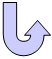Specifications

### Geometry Specifications

The recommended Finite Element Model uses four beam elements per arm.

 Length: L = 5000 mm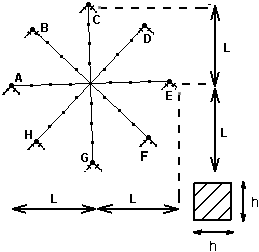Height: h = 125 mm

### Analysis Specifications

 Young Modulus (material): E = 200 GPa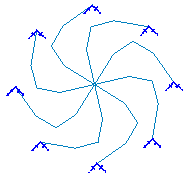Poisson's Ratio (material): ν = 0.3 Density: ρ = 8000 Kg/m3 Restraints: The structure is planar (all out-of plane motion is blocked). The eight ends of the double cross are simply-supported, hence the boundary conditions are: u = v = 0 at A, B, ... H.

##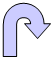Results

The table below presents the results of the 16 lowest natural frequencies of this structure:

 Mode Reference value [Hz] Computed result [Hz] Error [%] 1 11.336 11.336 0 2-3 17.687 17.687 0 4-8 17.715 17.715 0 9 45.477 45.477 0 10-11 57.364 57.364 0 12-16 57.683 57.683 0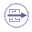To Perform the Test:

The DoubleCross.CATAnalysis document presents a complete analysis of this case.
Proceed as follow:

1. Open the CATAnalysis document.

2. Compute the case and generate an image called Deformed mesh.